This is “Multiplying Polynomials”, section 5.4 from the book Beginning Algebra (v. 1.0). For details on it (including licensing), click here.

Has this book helped you? Consider passing it on:
Creative Commons supports free culture from music to education. Their licenses helped make this book available to you.
DonorsChoose.org helps people like you help teachers fund their classroom projects, from art supplies to books to calculators.

## 5.4 Multiplying Polynomials

### Learning Objectives

1. Multiply a polynomial by a monomial.
2. Multiply a polynomial by a binomial.
3. Multiply a polynomial by any size polynomial.
4. Recognize and calculate special products.
5. Multiply polynomial functions.

## Multiplying by a Monomial

Recall the product rule for exponents: if m and n are positive integers, thenIn other words, when multiplying two expressions with the same base, add the exponents. This rule applies when multiplying a monomial by a monomial. To find the product of monomials, multiply the coefficients and add the exponents of variable factors with the same base. For example,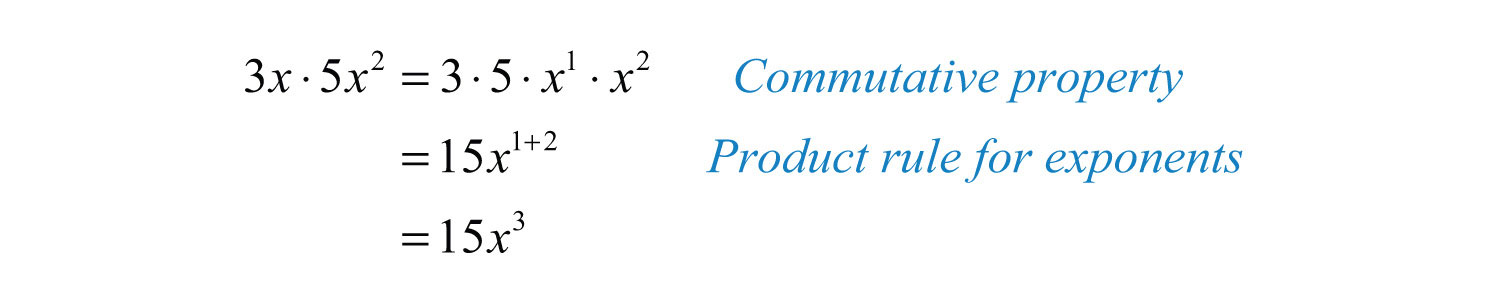To multiply a polynomial by a monomial, apply the distributive property and then simplify each term.

Example 1: Multiply: $−5x(4x−2)$.

Solution: In this case, multiply the monomial, $−5x$, by the binomial, $4x−2$. Apply the distributive property and then simplify.Answer: $−20x2+10x$

Example 2: Multiply: $2x2(3x2−5x+1)$.

Solution: Apply the distributive property and then simplify.Answer: $6x4−10x3+2x2$

Example 3: Multiply: $−3ab2(a2b3+2a3b−6ab−4)$.

Solution: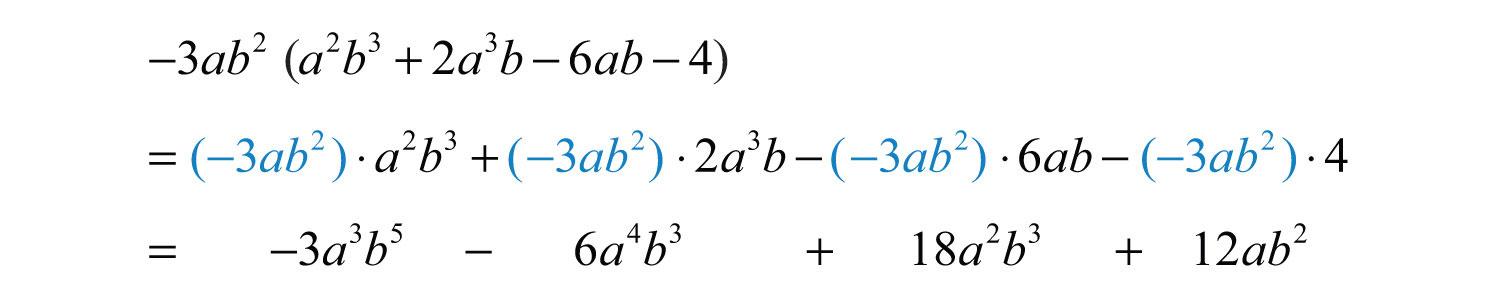Answer: $−3a3b5−6a4b3+18a2b3+12ab2$

To summarize, multiplying a polynomial by a monomial involves the distributive property and the product rule for exponents. Multiply all of the terms of the polynomial by the monomial. For each term, multiply the coefficients and add exponents of variables where the bases are the same.

Try this! Multiply: $−5x2y(2xy2−3xy+6x2y−1)$.

Answer: $−10x3y3+15x3y2−30x4y2+5x2y$

### Video Solution

(click to see video)

## Multiplying by a Binomial

In the same way that we used the distributive property to find the product of a monomial and a binomial, we will use it to to find the product of two binomials.Here we apply the distributive property multiple times to produce the final result. This same result is obtained in one step if we apply the distributive property to a and b separately as follows:This is often called the FOILWhen multiplying binomials we apply the distributive property multiple times in such a way as to multiply the first terms, outer terms, inner terms, and last terms. method. We add the products of the first terms of each binomial ac, the outer terms ad, the inner terms bc, and finally the last terms bd. This mnemonic device only works for products of binomials; hence it is best to just remember that the distributive property applies.

Example 4: Multiply: $(2x+3)(5x−2)$.

Solution: Distribute $2x$ and then distribute 3.Simplify by combining like terms.Answer: $10x2+11x−6$

Example 5: Multiply: $(12x−14)(12x+14)$.

Solution: Distribute $12x$ and then distribute $−14$.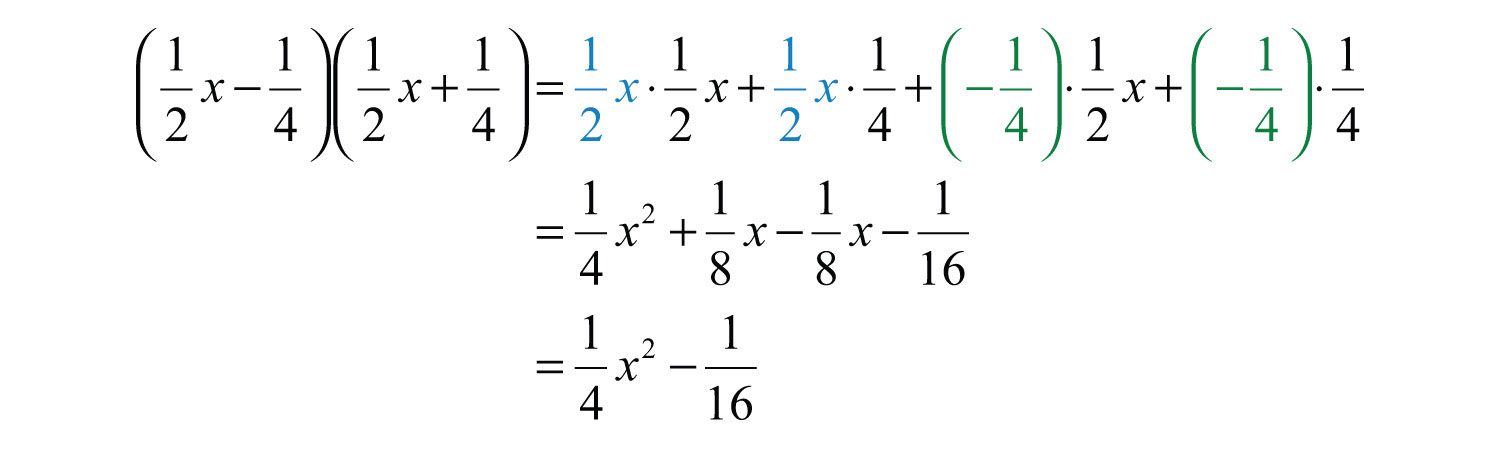Answer: $14x2−116$

Example 6: Multiply: $(3y2−1)(2y+1)$.

Solution:Answer: $6y3+3y2−2y−1$

After applying the distributive property, combine any like terms.

Example 7: Multiply: $(x2−5)(3x2−2x+2)$.

Solution: After multiplying each term of the trinomial by $x2$ and $−5$, simplify.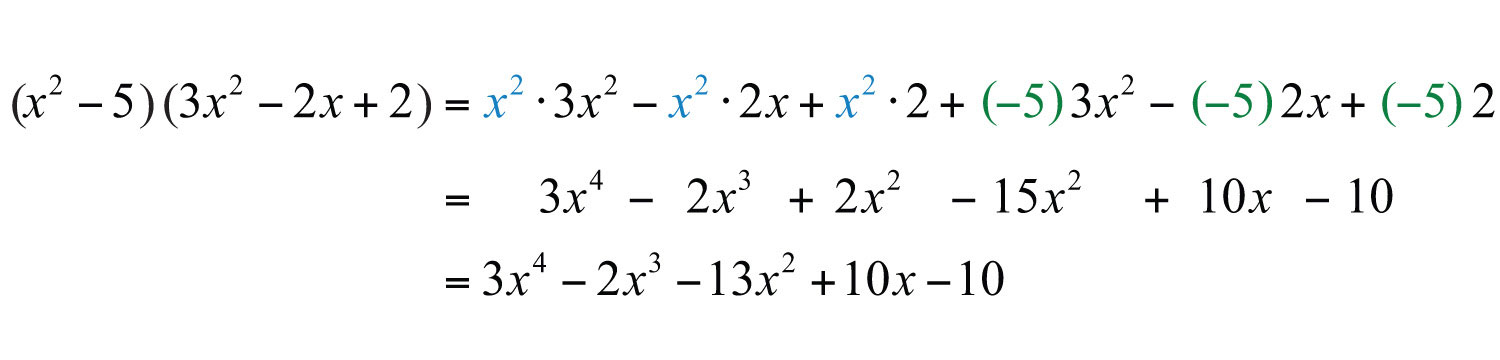Answer: $3x4−2x3−13x2+10x−10$

Example 8: Multiply: $(2x−1)3$.

Solution: Perform one product at a time.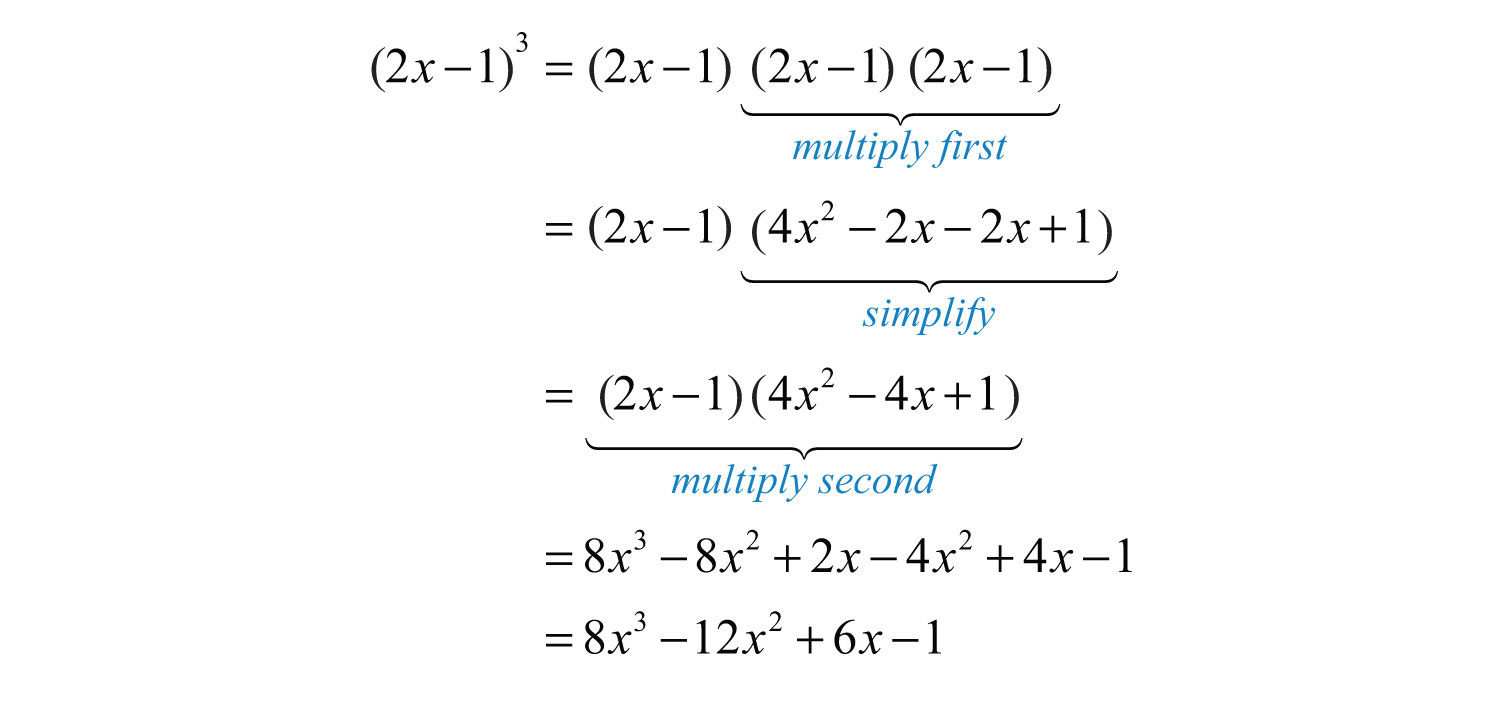Answer: $8x3−12x2+6x−1$

At this point, it is worth pointing out a common mistake:The confusion comes from the product to a power rule of exponents, where we apply the power to all factors. Since there are two terms within the parentheses, that rule does not apply. Care should be taken to understand what is different in the following two examples:Try this! Multiply: $(2x−3)(7x2−5x+4)$.

Answer: $14x3−31x2+23x−12$

### Video Solution

(click to see video)

## Product of Polynomials

When multiplying polynomials, we apply the distributive property many times. Multiply all of the terms of each polynomial and then combine like terms.

Example 9: Multiply: $(2x2+x−3)(x2−2x+5)$.

Solution: Multiply each term of the first trinomial by each term of the second trinomial and then combine like terms.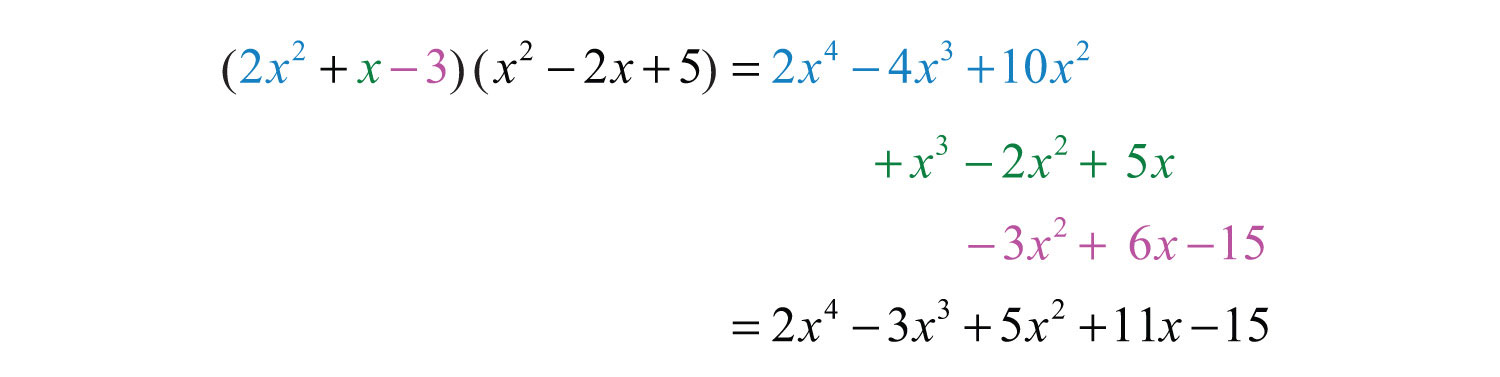Aligning like terms in columns, as we have here, aids in the simplification process.

Answer: $2x4−3x3+5x2+11x−15$

Notice that when multiplying a trinomial by a trinomial, we obtain nine terms before simplifying. In fact, when multiplying an n-term polynomial by an m-term polynomial, we will obtain n × m terms.

In the previous example, we were asked to multiply and found thatBecause it is easy to make a small calculation error, it is a good practice to trace through the steps mentally to verify that the operations were performed correctly. Alternatively, we can check by evaluatingWe can be fairly certain that we have multiplied the polynomials correctly if we check that a few values evaluate to the same results in the original expression and in the answer. any value for x in both expressions to verify that the results are the same. Here we choose x = 2: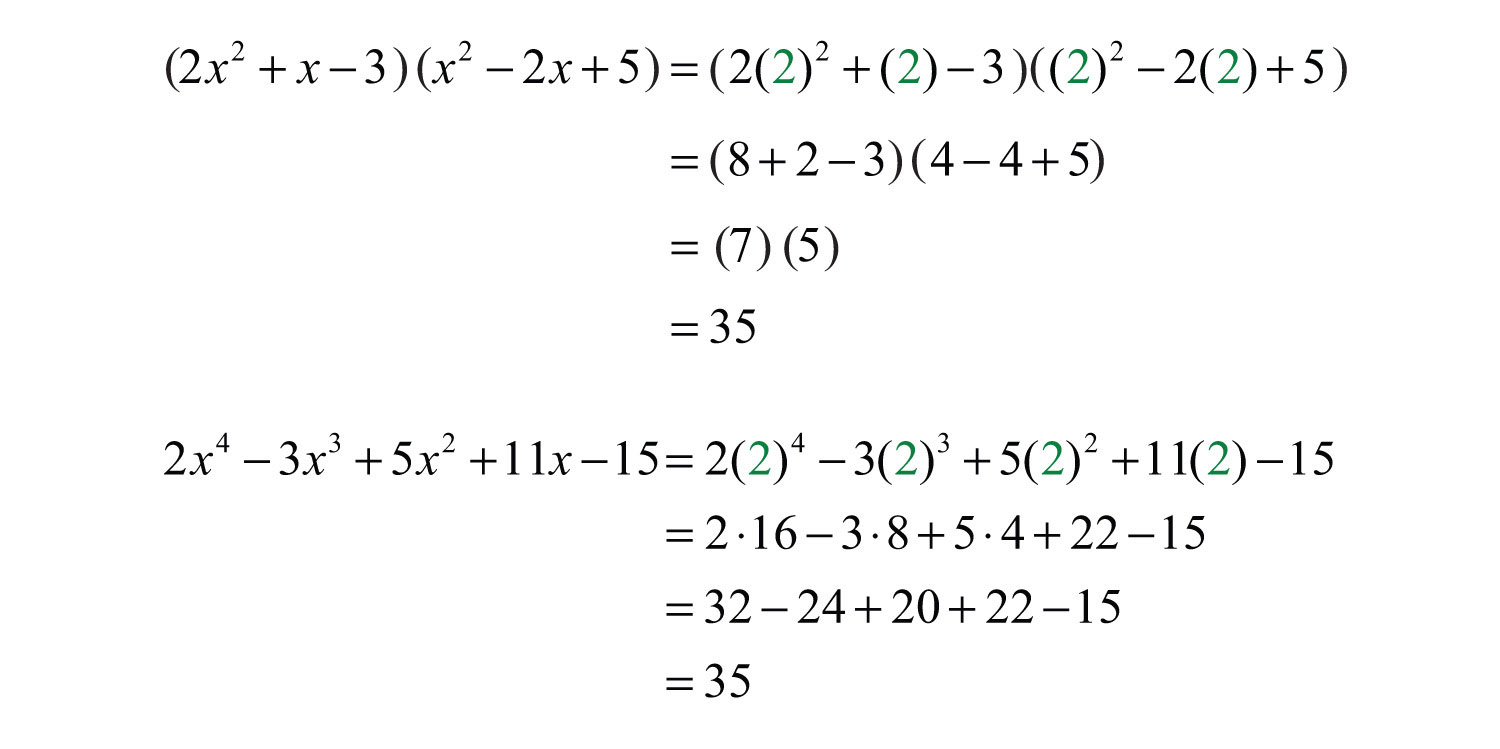Because the results could coincidentally be the same, a check by evaluating does not necessarily prove that we have multiplied correctly. However, after verifying a few values, we can be fairly confident that the product is correct.

Try this! Multiply: $(x2−2x−3)2$.

Answer: $x4−4x3−2x2+12x+9$

### Video Solution

(click to see video)

## Special Products

In this section, the goal is to recognize certain special products that occur often in our study of algebra. We will develop three formulas that will be very useful as we move along. The three should be memorized. We begin by considering the following two calculations: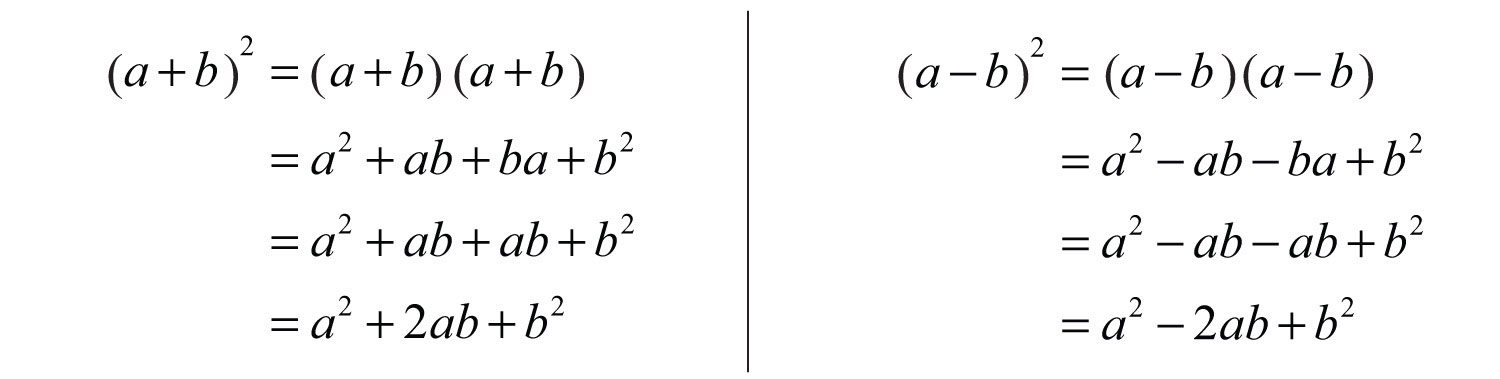This leads us to two formulas that describe perfect square trinomialsThe trinomials obtained by squaring the binomials $(a+b)2=a2+2ab+b2$ and $(a−b)2=a2−2ab+b2.$:We can use these formulas to quickly square a binomial.

Example 10: Multiply: $(3x+5)2$.

Solution: Here $a=3x$ and $b=5$. Apply the formula: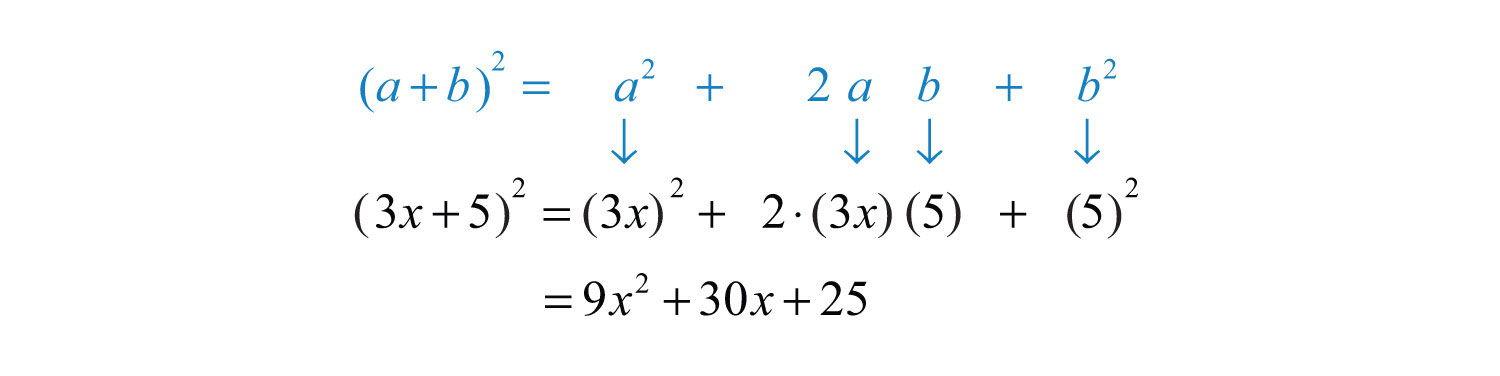Answer: $9x2+30x+25$

This process should become routine enough to be performed mentally.

Example 11: Multiply: $(x−4)2$.

Solution: Here $a=x$ and $b=4$. Apply the appropriate formula as follows:Answer: $x2−8x+16$

Our third special product follows:This product is called difference of squares$a2−b2=(a+b)(a−b),$ where a and b represent algebraic expressions.:The binomials $(a+b)$ and $(a−b)$ are called conjugate binomialsThe binomials $(a+b)$ and $(a−b)$.. Therefore, when conjugate binomials are multiplied, the middle term eliminates, and the product is itself a binomial.

Example 12: Multiply: $(7x+4)(7x−4)$.

Solution:Answer: $49x2−16$

Try this! Multiply: $(−5x+2)2$.

Answer: $25x2−20x+4$

### Video Solution

(click to see video)

## Multiplying Polynomial Functions

We use function notation to indicate multiplication as follows:

 Multiplication of functions: $(f⋅g)(x)=f(x)⋅g(x)$

Example 13: Calculate: $(f⋅g)(x)$, given $f(x)=5x2$ and $g(x)=−x2+2x−3$.

Solution: Multiply all terms of the trinomial by the monomial function $f(x)$.Answer: $(f⋅g)(x)=−5x4+10x3−15x2$

Example 14: Calculate: $(f⋅g)(−1)$, given $f(x)=−x+3$ and $g(x)=4x2−3x+6$.

Solution: First, determine $(f⋅g)(x)$.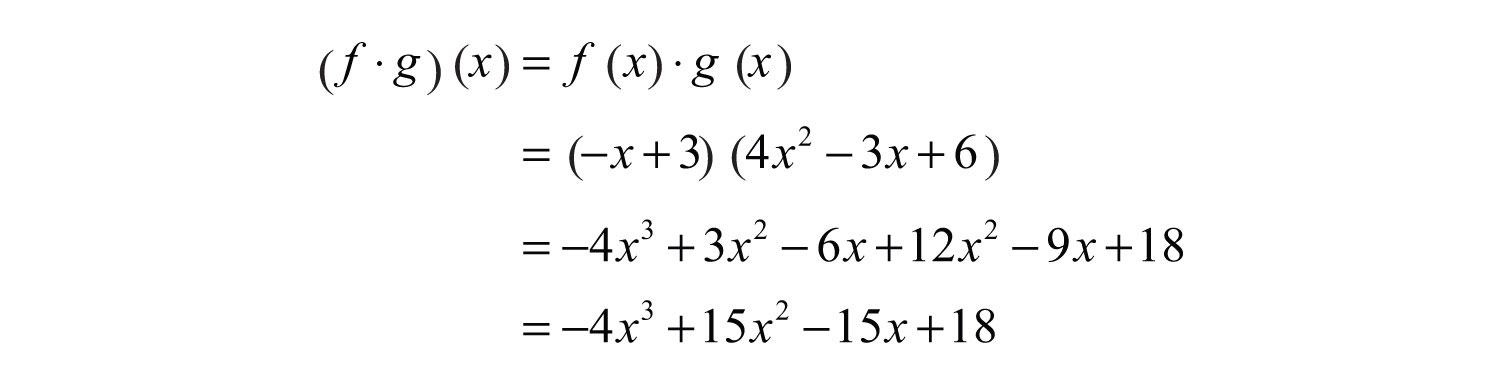We haveNext, substitute −1 for the variable x.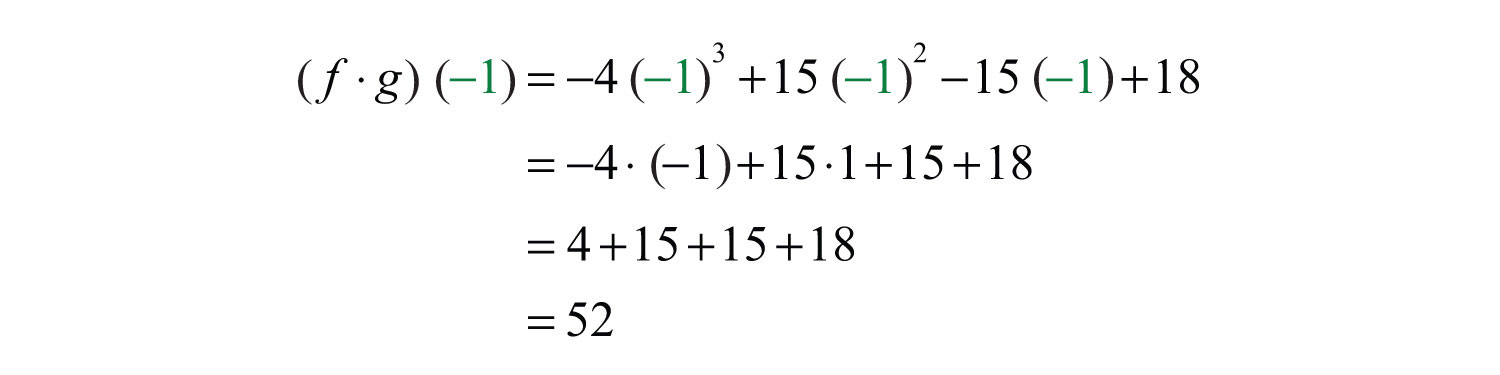Answer: $(f⋅g)(−1)=52$

Because $(f⋅g)(−1)=f(−1)⋅g(−1)$, we could alternatively calculate $f(−1)$ and $g(−1)$ separately and then multiply the results (try this as an exercise). However, if we were asked to evaluate multiple values for the function $(f⋅g)(x)$, it would be best to first determine the general form, as we have in the previous example.

### Key Takeaways

• To multiply a polynomial by a monomial, apply the distributive property and then simplify each of the resulting terms.
• To multiply polynomials, multiply each term in the first polynomial with each term in the second polynomial. Then combine like terms.
• The product of an n-term polynomial and an m-term polynomial results in an m × n term polynomial before like terms are combined.
• Check results by evaluating values in the original expression and in your answer to verify that the results are the same.
• Use the formulas for special products to quickly multiply binomials that occur often in algebra.

### Topic Exercises

Part A: Product of a Monomial and a Polynomial

Multiply.

1. $5x(−3x2y)$

2. $(−2x3y2)(−3xy4)$

3. $12(4x−3)$

4. $−34(23x−6)$

5. $3x(5x−2)$

6. $−4x(2x−1)$

7. $x2(3x+2)$

8. $−6x2(5x+3)$

9. $2ab(4a−2b)$

10. $5a2b(a2−b2)$

11. $6x2y3(−3x3y+xy2)$

12. $3ab3(−5ab3+6a2b)$

13. $−12x2y(4xy−10)$

14. $−3x4y2(3x8y3)$

15. $2x2(−5x3)(3x4)$

16. $4ab(a2b3c)(a4b2c4)$

17. $−2(5x2−3x+4)$

18. $45(25x2−50xy+5y2)$

19. $3x(5x2−2x+3)$

20. $−x(x2+x−1)$

21. $x2(3x2−5x−7)$

22. $x3(−4x2−7x+9)$

23. $14x4(8x3−2x2+12x−5)$

24. $−13x3(32x5−23x3+92x−1)$

25. $a2b(a2−3ab+b2)$

26. $6a2bc3(2a−3b+c2)$

27. $23xy2(9x3y−27xy+3xy3)$

28. $−3x2y2(12x2−10xy−6y2)$

29. Find the product of $3x$ and $2x2−3x+5$.

30. Find the product of $−8y$ and $y2−2y+12$.

31. Find the product of $−4x$ and $x4−3x3+2x2−7x+8$.

32. Find the product of $3xy2$ and $−2x2y+4xy−xy2$.

Part B: Product of a Binomial and a Polynomial

Multiply.

33. $(3x−2)(x+4)$

34. $(x+2)(x−3)$

35. $(x−1)(x+1)$

36. $(3x−1)(3x+1)$

37. $(2x−5)(x+3)$

38. $(5x−2)(3x+4)$

39. $(−3x+1)(x−1)$

40. $(x+5)(−x+1)$

41. $(y−23)(y+23)$

42. $(12x+13)(32x−23)$

43. $(34x+15)(14x+25)$

44. $(15x+310)(35x−52)$

45. $(y2−2)(y+2)$

46. $(y3−1)(y2+2)$

47. $(a2−b2)(a2+b2)$

48. $(a2−3b)2$

49. $(x−5)(2x2+3x+4)$

50. $(3x−1)(x2−4x+7)$

51. $(2x−3)(4x2+6x+9)$

52. $(5x+1)(25x2−5x+1)$

53. $(x−12)(3x2+4x−1)$

54. $(13x−14)(3x2+9x−3)$

55. $(x+3)3$

56. $(x−2)3$

57. $(3x−1)3$

58. $(2x+y)3$

59. $(5x−2)(2x3−4x2+3x−2)$

60. $(x2−2)(x3−2x2+x+1)$

Part C: Product of Polynomials

Multiply.

61. $(x2−x+1)(x2+2x+1)$

62. $(3x2−2x−1)(2x2+3x−4)$

63. $(2x2−3x+5)(x2+5x−1)$

64. $(a+b+c)(a−b−c)$

65. $(a+2b−c)2$

66. $(x+y+z)2$

67. $(x−3)4$

68. $(x+y)4$

69. Find the volume of a rectangular solid with sides measuring $x$, $x+2$, and $x+4$ units.

70. Find the volume of a cube where each side measures $x−5$ units.

Part D: Special Products

Multiply.

71. $(x+2)2$

72. $(x−3)2$

73. $(2x+5)2$

74. $(3x−7)2$

75. $(−x+2)2$

76. $(−9x+1)2$

77. $(a+6)2$

78. $(2a−3b)2$

79. $(23x+34)2$

80. $(12x−35)2$

81. $(x2+2)2$

82. $(x2+y2)2$

83. $(x+4)(x−4)$

84. $(2x+1)(2x−1)$

85. $(5x+3)(5x−3)$

86. $(15x−13)(15x+13)$

87. $(32x+25)(32x−25)$

88. $(2x−3y)(2x+3y)$

89. $(4x−y)(4x+y)$

90. $(a3−b3)(a3+b3)$

91. A box is made by cutting out the corners and folding up the edges of a square piece of cardboard. A template for a cardboard box with a height of 2 inches is given. Find a formula for the volume, if the initial piece of cardboard is a square with sides measuring x inches.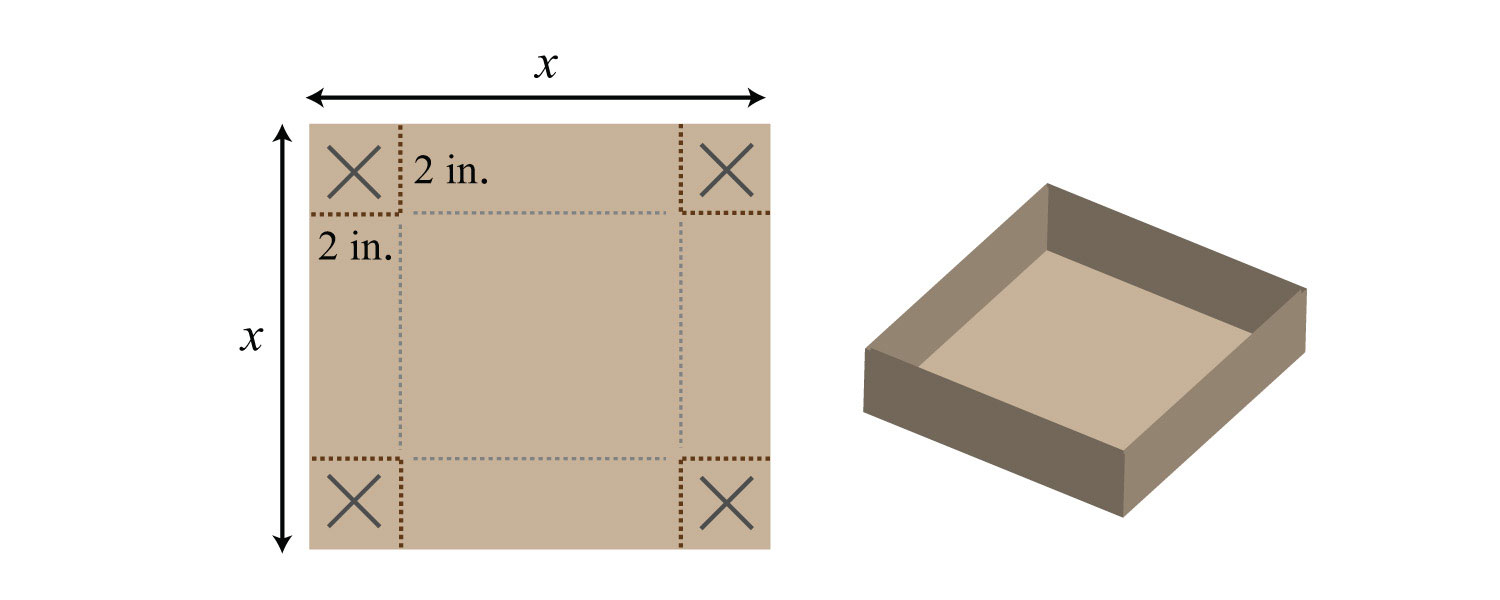92. A template for a cardboard box with a height of x inches is given. Find a formula for the volume, if the initial piece of cardboard is a square with sides measuring 12 inches.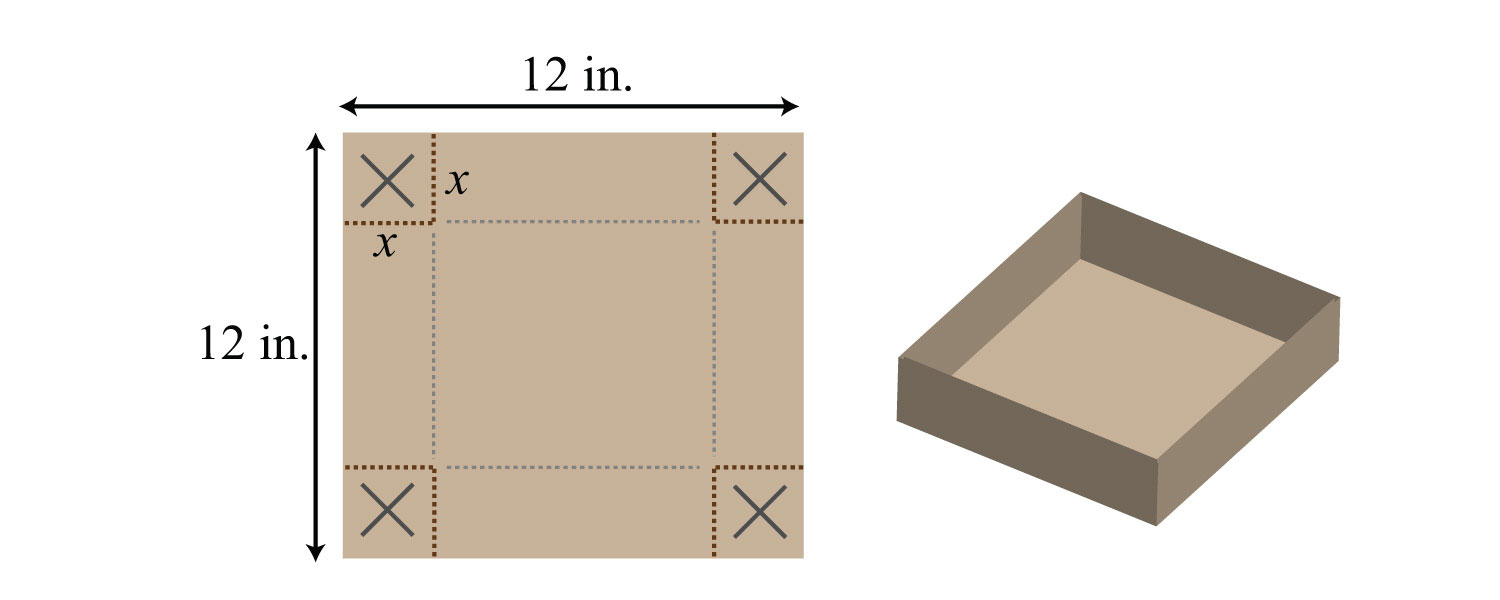Part E: Multiplying Polynomial Functions

For each problem, calculate $(f⋅g)(x)$, given the functions.

93. $f(x)=8x$ and $g(x)=3x−5$

94. $f(x)=x2$ and $g(x)=−5x+1$

95. $f(x)=x−7$ and $g(x)=6x−1$

96. $f(x)=5x+3$ and $g(x)=x2+2x−3$

97. $f(x)=x2+6x−3$ and $g(x)=2x2−3x+5$

98. $f(x)=3x2−x+1$ and $g(x)=−x2+2x−1$

Given $f(x)=2x−3$ and $g(x)=3x−1$, find the following.

99. $(f⋅g)(x)$

100. $(g⋅f)(x)$

101. $(f⋅g)(0)$

102. $(f⋅g)(−1)$

103. $(f⋅g)(1)$

104. $(f⋅g)(12)$

Given $f(x)=5x−1$ and $g(x)=2x2−4x+5$, find the following.

105. $(f⋅g)(x)$

106. $(g⋅f)(x)$

107. $(f⋅g)(0)$

108. $(f⋅g)(−1)$

109. $(f⋅g)(1)$

110. $(f⋅g)(12)$

111. $(f⋅f)(x)$

112. $(g⋅g)(x)$

Part F: Discussion Board Topics

113. Explain why $(x+y)2≠x2+y2$.

114. Explain how to quickly multiply a binomial with its conjugate. Give an example.

115. What are the advantages and disadvantages of using the mnemonic device FOIL?

1: $−15x3y$

3: $2x−32$

5: $15x2−6x$

7: $3x3+2x2$

9: $8a2b−4ab2$

11: $−18x5y4+6x3y5$

13: $−2x3y2+5x2y$

15: $−30x9$

17: $−10x2+6x−8$

19: $15x3−6x2+9x$

21: $3x4−5x3−7x2$

23: $2x7−12x6+18x5−54x4$

25: $a4b−3a3b2+a2b3$

27: $6x4y3−18x2y3+2x2y5$

29: $6x3−9x2+15x$

31: $−4x5+12x4−8x3+28x2−32x$

33: $3x2+10x−8$

35: $x2−1$

37: $2x2+x−15$

39: $−3x2+4x−1$

41: $y2−49$

43: $316x2+720x+225$

45: $y3+2y2−2y−4$

47: $a4−b4$

49: $2x3−7x2−11x−20$

51: $8x3−27$

53: $3x3+52x2−3x+12$

55: $x3+9x2+27x+27$

57: $27x3−27x2+9x−1$

59: $10x4−24x3+23x2−16x+4$

61: $x4+x3+x+1$

63: $2x4+7x3−12x2+28x−5$

65: $a2+4ab−2ac+4b2−4bc+c2$

67: $x4−12x3+54x2−108x+81$

69: $x3+6x2+8x$

71: $x2+4x+4$

73: $4x2+20x+25$

75: $x2−4x+4$

77: $a2+12a+36$

79: $49x2+x+916$

81: $x4+4x2+4$

83: $x2−16$

85: $25x2−9$

87: $94x2−425$

89: $16x2−y2$

91: $V=2x2−16x+32$ cubic inches

93: $(f⋅g)(x)=24x2−40x$

95: $(f⋅g)(x)=6x2−43x+7$

97: $(f⋅g)(x)=2x4+9x3−19x2+39x−15$

99: $(f⋅g)(x)=6x2−11x+3$

101: $(f⋅g)(0)=3$

103: $(f⋅g)(1)=−2$

105: $(f⋅g)(x)=10x3−22x2+29x−5$

107: $(f⋅g)(0)=−5$

109: $(f⋅g)(1)=12$

111: $(f⋅f)(x)=25x2−10x+1$